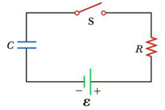Chapter 18, Problem 35P

Chapter
Section
Textbook Problem

Consider a series RC circuit as in Figure P18.35 for which R = 1.00 MΩ, C = 5.00 µF, and ε = 30.0 V. Find (a) the time constant of the circuit and (b) the maximum charge on the capacitor after the switch is thrown closed. (c) Find the current in the resistor 10.0 s after the switch is closed.Figure P18.35 Problem 35 and 38.

(a)

To determine
The time constant τ of the circuit.

Explanation

Given Info: Resistance R is 1.00 , Capacitance C is 5.00μF .

Explanation:

Formula to calculate time constant τ is

τ=RC

• τ is the time constant,
• R is the Resistance,
• C is the capacitance,

Substitute 1.00 for R, 5.00μF for C, to find τ

τ=1

(b)

To determine
The maximum charge on the capacitor Qmax after the switch is thrown closed

(c)

To determine
The current in the resistor 10.0 s after the switch is closed.

Still sussing out bartleby?

Check out a sample textbook solution.

See a sample solution

The Solution to Your Study Problems

Bartleby provides explanations to thousands of textbook problems written by our experts, many with advanced degrees!

Get Started

Find more solutions based on key concepts